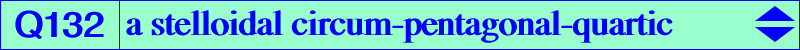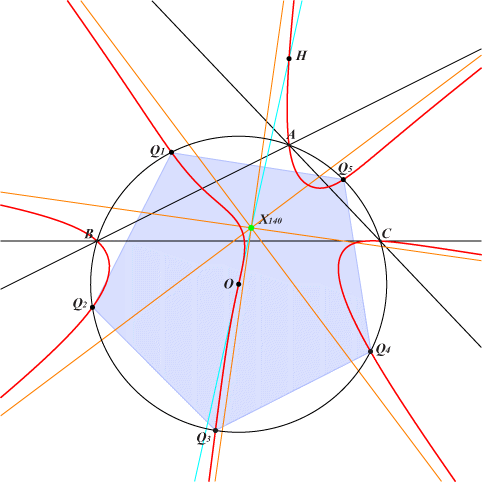too complicated to be written here. Click on the link to download a text file.X(3), X(4) anti-points, see Table 77See Q125 for general explanations where Q132 is Q(X30). The radial center is X(140). The tangent at X(3) is the Euler line and X(3) is a point of inflexion. Q132 has the remarkable property of meeting the circumcircle at A, B, C and five other points which are the vertices of a regular pentagon. *** More generally, any quartic Q(P) with P at infinity is a similar "pentagonal" quartic. The radial center is always X(140). The tangent tgO at X(3) to Q(P) passes through P and meets Q(P) at two other (real or not) points M1, M2 which are isogonal conjugates hence on the McCay cubic K003. Since Q(P) is a curve of class 12, eleven other tangents can be drawn from P to Q(P). When P traverses the line at infinity, the locus of contacts of these tangents is the stelloidal cubic K910. The points of inflexion of Q(P) lie on a complicated 10th degree curve passing through A, B, C, X(3), X(15), X(16), the vertices of the Thomson triangle, the (quadruple) circular points at infinity. Q133 = Q(X952) and Q134 = Q(X517) are other examples of "pentagonal" quartics.Other related loci Let tgO, cpO, stO (resp. tgP, cpP, stP) be the 1st, 2nd, 3rd polar curves of O = X(3) (resp. P) in Q(P). • tgO is the line OP and tgP is a line passing through X(140). • cpO and cpP are rectangular hyperbolas with centers X(7575) and X(140) respectively. cpO passes through O and X(23) whose midpoint is X(7575). • stO is a stelloidal cubic with radial center the centroid of {O, X{23}, X(323)}, also the inverse of X(5609) in (O). stP is K910 and is independent of P.   tgO, cpO, stO meet Q(P) on K003, Q136, Q037 respectively. cpO and stO meet at six points namely O (counted twice since the tangent is the same) and four other points on the focal cubic K912. These curves are represented in the figure below.tgP and cpP meet on a central circular quintic with center X(140). This quintic has three real asymptotes parallel to those of the McCay cubic K003. The following table gives the loci of common points of tgP and cpP with curves passing through O and Q(P). All these curves are stelloids of various degree and pass through X(3). ∞K003 denotes the infinite points of K003.tgP cpP tgO nodal cubic through X(3), X(140), X(1385), X(13334), ∞K003 the homothetic of K028 under h(X631, –1/4) radial center : X(12100) cubic through X(3), X(5), ∞K003 radial center : X(549) cpO quintic through X(3), X(23), X(140) radial center S1 on the Euler line quartic through X(3), X(5), X(23) radial center S2 on the Euler line stO septic through X(3), X(140) radial center S3 on the line X(548), X(1511) quintic through X(3) radial center S4 on the line X(5), X(1511) Q(P) nonic through X(3), X(140), ∞K003 radial center : X(140) sextic through X(3), ∞K003, ∞K024 radial center : X(140)Additional properties by Peter Moses (2017-07-14) -------------------------------------------------------------------------------------------------------- S1 on line {2, 3} 6 X - X, 9 X - 4 X, 3 X + 2 X, 8 X - 3 X, X + 4 X, X + 9 X, X - 6 X, X - 6 X, 2 X + 3 X. -------------------------------------------------------------------------------------------------------- S2 on lines {2, 3}, {3292, 11694}, {11561, 13392}, {11695, 13365}. Midpoint of X (i) and X (j) for these {i, j} : {140, 7575}, {546, 10295}, {548, 11799}, {7426, 12100}. X + 3 X, X + 3 X, 3 X - X, 5 X + 3 X, 3 X - X, 3 X - 11 X, 9 X - X, 5 X - X, X + 3 X, X + 3 X, 2 X - 3 X, 5 X - X, 15 X + X, X + 3 X, X - 3 X, 3 X - X, X + 2 X, 5 X + 3 X. {X (i), X (j)} - harmonic conjugate of X (k) for these (i, j, k) : (140, 12100, 7496), (548, 10096, 11799). -------------------------------------------------------------------------------------------------------- S3 on lines {110, 12100}, {140, 3448}, {546, 10733}, {547, 5972}, {548, 1511}, {5642, 12101}, {12383, 12812}. X - 8 X, 9 X - 2 X, 9 X - 16 X, 11 X - 4 X, 2 X - 9 X, X - 8 X, 15 X - 8 X, 10 X - 3 X, 15 X - X, 4 X + 3 X, 8 X - X, 2 X + 5 X, X - 8 X. -------------------------------------------------------------------------------------------------------- S4 on lines {3, 13392}, {5, 1511}, {110, 549}, {125, 11539}, {140, 3448}, {399, 3530}, {547, 12902}, {550, 7728}, {3524, 12308}, {3628, 12383}, {3845, 12121}, {3857, 10733}, {5642, 8703}, {7471, 11749}, {9143, 11812}, {10283, 12778}, {10620, 12100}. 2 X + 3 X, X + 4 X, 6 X - X, X + 4 X, 3 X - 8 X, 3 X + 2 X, 13 X - 8 X, 13 X - 3 X, 13 X + 2 X, 3 X + 2 X, 4 X + X, 18 X - 13 X, 9 X - 4 X, 6 X - X, 9 X + X, X - 6 X, X + 4 X, 7 X - 2 X, 4 X - 9 X, X + 4 X, X + 9 X, X - 6 X, X + 4 X, 4 X + X, X + 4 X, X - 6 X, 3 X + 2 X, 9 X + X, 4 X + X, 3 X + 2 X, 11 X - 16 X, 11 X - 6 X, 11 X + 4 X, 6 X - X, X + 4 X.